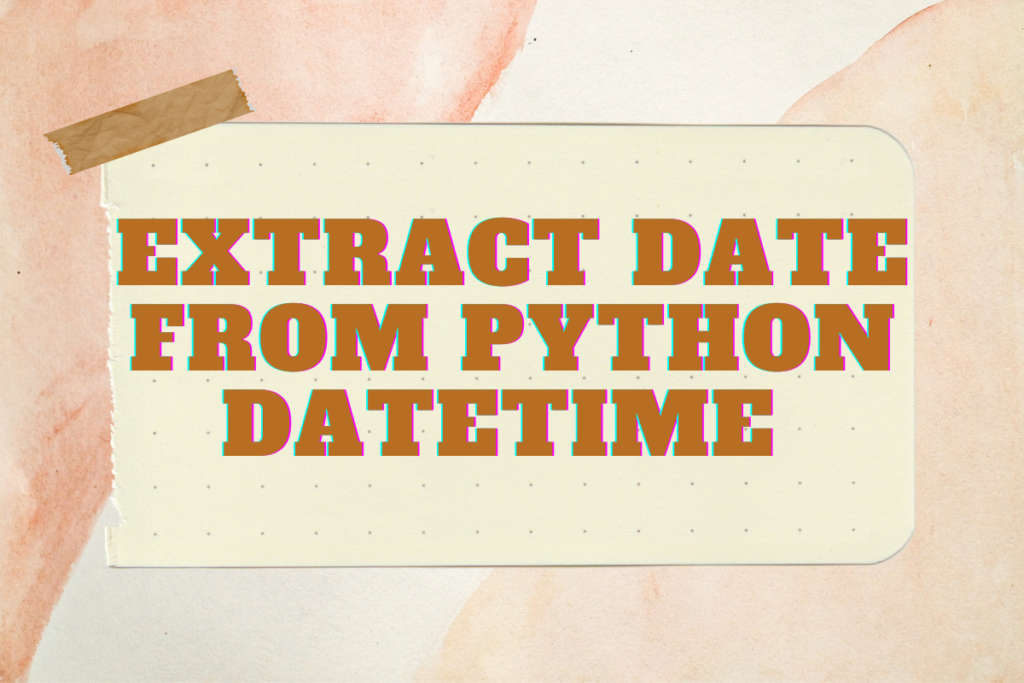# Extracting Date from Datetime in Python: 3 Methods ExplainedDatetime makes us to identify in great details elements like hours, minutes, seconds, dates, days of the month, week, years, etc. It is very effective way of handling the date and time related data in Python. In this article, we shall explore the following techniques that could be used to extract only the date from a python datetime.

• Using the strftime( ) function
• Using the %s operator
• Using the date( ) function

In Python, you can extract date from datetime using three methods: the strftime() function, the %s operator, and the date() function. The strftime() function uses format codes to convert datetime into a string representation. The %s operator allows for simple extraction of date elements, while the date() function directly returns the current date from the datetime object. Each method offers a different approach to handling datetime data and extracting the date information.

## Method 1: Extracting Date with strftime() Function

The strftime() method considers one or more format codes as an argument and it shall strip out a formatted string based on the format code selected. To put in simple terms the date and time objects are being converted into their equivalent string representation. Following are some of the different format codes that could be used in this function,

```%Y, %m, %d
```

This is not to be confused with the strptime( ) function available in the datetime module that is used for the conversion of the time stamp which is given as an input string into a date-time object.

Now we shall put into practice the format codes by applying them to strip off only the date details from the datetime function in Python. Here is a stepwise walkthrough on the extraction of the date details using the strftime( ) function.

```from datetime import datetime

localcurrentdateandtime = datetime.now() # Get the local date and time
print("Local current date and time:",localcurrentdateandtime) # print the local date and time

currentyear = localcurrentdateandtime.strftime("%Y") # Get the current year from the local date and time
print("Getting the year:", currentyear) # print the year from the local date and time

currentmonth = localcurrentdateandtime.strftime("%m") # Get the current month from the local dateand time
print("Getting the month:", currentmonth) # print the month from the local date and time

currentday = localcurrentdateandtime.strftime("%d") # Get the current day from the local date and time
print("Getting the day:", currentday) # print the day from the local date and time

currentdatetime = localcurrentdateandtime.strftime("%m/%d/%Y") # Get the current date from the local date and time
print("Getting date:",currentdatetime) # print the date from the local date and time
```

## Method 2: Extracting Date Using %s Operator

Another technique to get the date only extracted from the datetime is the %s operator. It is a fairly simple approach which starts off by importing the datetime library as shown below.

```from datetime import datetime
```

Following that one shall now get on with setting the input using the datetime( ) function.

```cdt = datetime.now() # Get the local date and time
print("Local current date and time:",cdt) # print the local date and time
```

Now it is time to use the %s operator to extract only the date from the above.

```op = '%s/%s/%s' % (cdt.month, cdt.day, cdt.year)
print ("Extracted date:", op)
```

## Method 3: Extracting Date with date() Function

This is one of the simplest techniques that could be deployed to extract only the date from the datetime in Python. The date() function method is used to return the current date from the datetime in Python. But the worthwhile stuff is that it can be done in a jiffy! Here is how.

One shall get things started by importing the datetime module, followed by declaring a variable to be fed as an input with the current date.

```import datetime
cdt = datetime.today() # Create the current datetime
print ("Current date & time:", cdt) # Print the current datetime
```

Then one shall move on towards tagging this input along with the date( ) function to extract only the date details as shown below.

```currentdate = cdt.date() # Using the date( ) function to get only the current date from current datetime
print("Current date:", currentdate)# Print only the current date
```